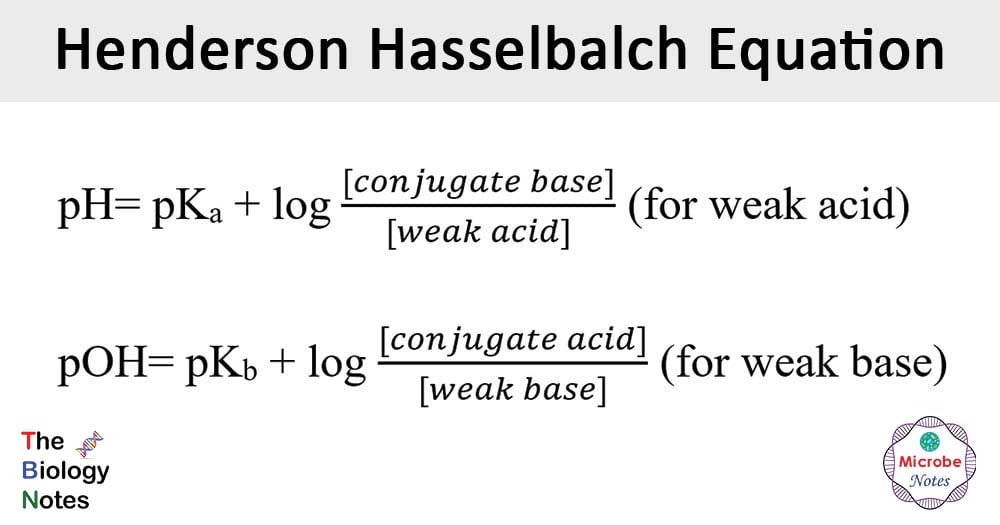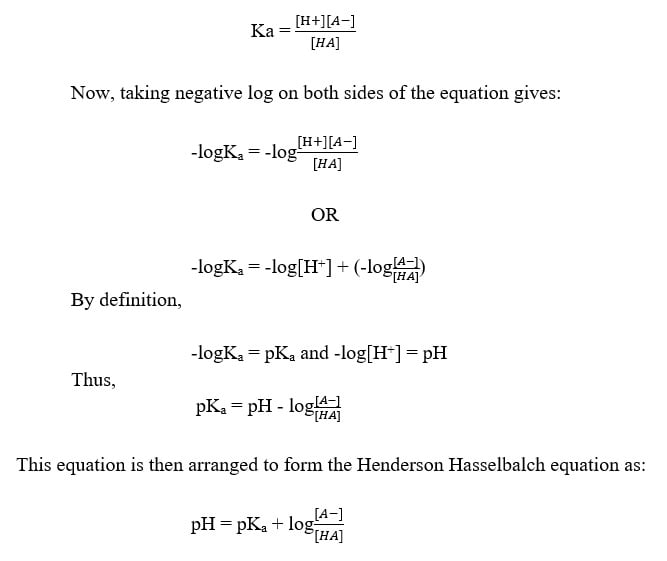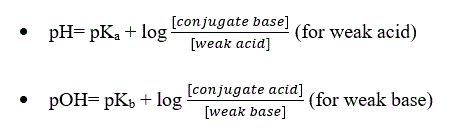# Henderson Hasselbalch Equation

Watch Biology Educational Videos

Last Updated on May 23, 2020 by Sagar Aryal

## Henderson Hasselbalch Equation DefinitionThe Henderson-Hasselbalch equation is the equation commonly used in chemistry and biology to determine the pH of a solution.

• This equation shows a relationship between the pH or pOH of the solution, the pKa or pKb, and the concentration of the chemical species involved.
• This equation was developed independently by the American biological chemist L. J. Henderson and the Swedish physiologist K. A. Hasselbalch to determine the pH of the bicarbonate buffer system in blood.
• This type of kinetic analysis has enabled us for nearly a century to relate theoretically the changes of the acidic intensity of dilute solutions to a quantity of acid or base added or subtracted.
• This equation can be considered as the backbone of acid-base physiology.
• This equation is commonly used to determine the amount of acid and conjugate base required to prepare a buffer of the desired pH.

## Objectives of Henderson Hasselbalch Equation

The principal objectives of the Henderson Hasselbalch equation include the following:

1. To calculate the pH, pOH, [H3O+ ]tot, [OH- ]tot, [H3O+ ]water, and [OH- ]water in a solution containing a strong acid (base) given the initial concentration of the acid (base).
2. To describe how a buffer solution (either acidic or basic) can resist significant changes in pH when small amounts of either acid or base are added to the buffer solution.
3. To describe how either an acidic or basic buffer solution is prepared.
4. To describe a “buffer solution”.
5. To describe “buffer capacity”.
6. To determine whether an aqueous solution of salt will be acidic, basic, or neutral given values of Ka and Kb for conjugate acid-base pairs.
7. To describe how the relative strengths of the conjugate acids or bases can be evaluated using the values of Kb and Ka for the bases and acids, respectively.
8. To determine the protonation state of different biomolecule functional groups in a pH seven buffer.

## Principle/Theory/Derivation of Henderson Hasselbalch Equation

• According to the Bronsted-Lowry theory of acids and bases, an acid (HA) can donate a proton (H+) while a base (B) can accept a proton.
• An acid after losing a proton forms a conjugate base (A), and the protonated base exists as conjugate acid (BH+).
• The dissociation of acid is expressed in terms of the equilibrium equation as:

HA      ↔       H+  A

• This relationship can be described in terms of the equilibrium constant as:## Equation

• The Henderson Hasselbalch equation can be expressed in two ways:where pKa= dissociation constant of acid

pKb= dissociation constant of base

## Applications of Henderson Hasselbalch Equation

### Calculating the pH of a solution using pKa

• This equation can be used to determine the pH of different solutions in different chemical equations as well as in biological systems like enzymes and proteins.

### Calculating the ionized and unionized concentrations of chemicals.

• One of the most potent applications of the Henderson Hasselbalch equation is the ability to determine the concentrations of ionized and unionized chemicals.
• Generally, the amount of ionized and unionized species is detected using some spectroscopic technique, and thus, this equation is useful in the condition where spectroscopic studies are not feasible.
• The knowledge of the concentration of ionized and unionized chemicals is essential in fields like organic chemistry, analytical chemistry, and pharmaceuticals sciences,

### Calculating pKa of a molecule using pH

• Determining the pKa of a molecule is important as the pKa is an essential characteristic of the chemistry of the structure of the molecule.
• Henderson Hasselbalch equation can be used to determine the pKa when the ratio of ionized and unionized forms and the pH of the solution is known.

### Determination of solubility

• It has been observed that the Henderson Hasselbalch equation is important in determining the pH dependency of solubility.
• Based on the pH of a solution, the solubility of the solution can be determined, and there is also a close relationship between pH and solubility of various components in a solution.

### Calculating the isoelectric point of protein

• This equation can also be used in determining the isoelectric point of different proteins (pH at which proteins neither lose or accept protons).

## Limitations

• The most critical assumption of this equation is that the concentration of acid and its conjugate base will remain the same during the equilibrium.
• The significance of hydrolysis of water and its effect on the pH of the overall solution is neglected.
• Similarly, the hydrolysis of the base and dissociation of acid is also neglected.
• The assumption made in the equation might fail while dealing with strong acids or bases.

This site uses Akismet to reduce spam. Learn how your comment data is processed.